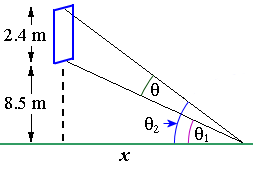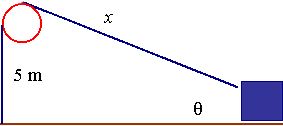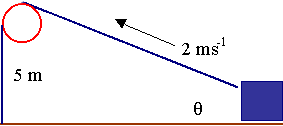Search IntMath
Close

450+ Math Lessons written by Math Professors and Teachers

5 Million+ Students Helped Each Year

1200+ Articles Written by Math Educators and Enthusiasts

Simplifying and Teaching Math for Over 23 Years

# 4. Applications: Derivatives of Trigonometric Functions

by M. Bourne

We can now use derivatives of trigonometric and inverse trigonometric functions to solve various types of problems.

### Example 1

Find the equation of the normal to the curve of y=tan^-1(x/2) at x=3.

If you need a refresher, see the section on Tangent and Normals.

The derivative of y=arctan(u) is given by:

(dy)/(du)=1/(1+(u)^2)

In this example, we have u=x/2, so the derivative is:

(dy)/(dx)=1/(1+(x/2)^2)(1/2)

When x = 3, this expression is equal to: 0.153846

So the slope of the tangent at x = 3 is 0.153846.

The slope of the normal at x = 3 is given by:

(-1)/0.153846=-6.5

So the equation of the normal is given by:

(When x = 3, y = 0.9828)

y − 0.9828 = -6.5(x − 3)

That is,

y = -6.5x + 20.483

Here is the graph of our situation:

The graph of y=arctan(x/2) showing the tangent and the normal at x=3.

### Example 2

The apparent power Pa of an electric circuit whose power is P and whose impedance phase angle is θ, is given by

P_a = P sec θ.

Given that P is constant at 12 W, find the time rate of change of Pa if θ is changing at the rate of 0.050 rad/min, when θ = 40°.

Using chain rule, we have:

(dP_a)/(dt)=(dP_a)/(d theta)(d theta)/(dt)

Now P_a= P sec θ = 12 sec θ (since P = 12\ "W")

(dP_a)/(d theta)=12 sec theta tan theta

We are told

(d theta)/(dt)=0.050

So

(dP_a)/(dt)=(dP_a)/(d theta)(d theta)/(dt)

=(12 sec theta tan theta)(0.050)

=0.6 sec theta tan theta

When θ = 40°, this expression is equal to: 0.657 W/min.

### Example 3

A machine is programmed to move an etching tool such that the position of the tool is given by x = 2 cos 3t and y = cos 2t, where the dimensions are in cm and time is in s. Find the velocity of the tool for t = 4.1 s.

v_x=(dx)/(dt)=-6 sin 3t

v_y=(dy)/(dt)=-2 sin 2t

At t = 4.1, v_x = 1.579 and v_y = -1.88.

So

v=sqrt((v_x)^2+(v_y)^2)

=sqrt((1.579)^2+(-1.88)^2)

=2.46\ "cm"//s

For velocity, we need to also indicate direction. First, we find the appropriate acute angle (the "reference" angle):

alpha=tan^-1(1.88 / 1.579)=50^@

So since we are in the 4th quadrant when v_x is positive and v_y is negative, the required angle is 360^@ - 50^@ = 310^@.

(See the following for background on how to find this angle: Trigonometric Functions of any Angle.)

### Example 4

The television screen at a sports arena is vertical and 2.4 m high. The lower edge is 8.5 m above an observer's eye level. If the best view of the screen is obtained when the angle subtended by the screen at eye level is a maximum, how far from directly below the screen must the observer be?

(Diagam not to scale)We define θ1 and θ2 as shown in the diagram such that:

θ = θ_2 - θ_1.

Let x be the distance from directly under the screen to the observer. To maximise θ, we will need to find

dy/dx

and then set it to 0.

We note that

tan theta_1=8.5/x, and

tan theta_2=10.9/x

This gives:

theta_1=tan^-1(8.5)/x  and

theta_2=tan^(-1) 10.9/x

Now since θ = θ_2 - θ_1,

theta=tan^-1 10.9/x-tan^-1 8.5/x

We have a function of a function in each term.

Now, in the first term, if we let

u=10.9/x=10.9x^-1

then

(du)/(dx)=d/(dx)(10.9x^-1)

=-10.9x^-2

=-10.9/x^2

Similarly for the second term, we will have:

d/(dx)(8.5x^-1)

=-8.5x^-2

=-8.5/x^2

So we have:

(d theta)/(dx)=1/(1+((10.9)/x)^2)(-10.9/x^2) -1/(1+((8.5)/x)^2)(-8.5/x^2)

=1/x^2((-10.9)/(1+(10.9^2)/(x^2))+8.5/(1+(8.5^2)/(x^2)))

Next, we multiply the x2 in the denominator (bottom) of the first fraction by the denominators of the 2 fractions in brackets, giving:

=(-10.9)/(x^2+10.9^2)+8.5/(x^2+8.5^2)

=(-10.9(x^2+8.5^2)+8.5(x^2+10.9^2))/((x^2+10.9^2)(x^2+8.5^2))

=(-2.4x^2+222.36)/((x^2+10.9^2)(x^2+8.5^2))

To find when this equals 0, we need only determine when the numerator (the top) is 0.

That is

-2.4x^2 + 222.36 = 0

This occurs when x = 9.63 (we take positive case only)

So the observer must be 9.63\ "m" from directly below the screen to get the best view.

### Example 5

A winch on a loading dock is used to drag a container along the ground. The winch winds the cable in at 2ms-1 and is 5 m above the ground. At what rate is the angle θ between the cable and the ground changing when 10 m of cable is out?

Scalar Diagram (involving distances only):We can see that:

sin theta=5/x, so

theta=sin^-1(5/x)

Vector Diagram (involving velocities):[See the section on Vector concepts for more on vectors and scalars.]

We are also given that:

(dx)/(dt)=-2\ "ms"^-1

Since we need (d theta)/(dt), we use:

(d theta)/(dt)=(d theta)/(dx)(dx)/(dt)

Because

theta=sin^-1(5/x)

we have:

(d theta)/(dx)=1/(sqrt(1-(5/x)^2))(d(5/x))/(dx)

=(-5)/(x^2sqrt(1-(5/x)^2))

So

(d theta)/(dt)=(d theta)/(dx)(dx)/(dt)

=(-5)/(x^2sqrt(1-(5/x)^2))(-2)

=10/(x^2sqrt(1-(5/x)^2))

We want to know the rate of change of θ when x = 10 m, so we substitute, as follows:

(d theta)/(dt)=10/(10^2sqrt(1-(5/10)^2))

=0.1155\ "rad"//"s"

## Problem SolverThis tool combines the power of mathematical computation engine that excels at solving mathematical formulas with the power of GPT large language models to parse and generate natural language. This creates math problem solver thats more accurate than ChatGPT, more flexible than a calculator, and faster answers than a human tutor. Learn More.